﻿ Study of Electrocapillarity in Dielectrics Using Palm Oil

### Study of Electrocapillarity in Dielectrics Using Palm Oil

Nwodo A. N, Ugwu E.IOPEN ACCESSPEER-REVIEWED

## Study of Electrocapillarity in Dielectrics Using Palm Oil

Nwodo A. N1, Ugwu E.I2,1Department. of Physics, Federal University, Ndufu-Alike – Ikwo,

2Department of Industrial Physics, Ebonyi State University, P.B.M 53, Abakaliki, Nigeria

### Abstract

This paper presents analytical and experimental study of electrocapillarity in dielectrics under the frame work of parallel plates using palm oil.. First, Mathematical approach was used to derive an expression relating capillary ascent with the applied field. The results obtained from the experiment and that of the analytical results were compared with the expected results from the equation relating the liquid ascent and the applied field obtained from Lippmann equation, where it was observed that there is no evidence of linear relation between the height of the capillary rise of oil in the tube and the applied field.

### At a glance: Figures

1
Prev Next

• N, Nwodo A., and Ugwu E.I. "Study of Electrocapillarity in Dielectrics Using Palm Oil." International Journal of Physics 2.3 (2014): 83-85.
• N, N. A. , & E.I, U. (2014). Study of Electrocapillarity in Dielectrics Using Palm Oil. International Journal of Physics, 2(3), 83-85.
• N, Nwodo A., and Ugwu E.I. "Study of Electrocapillarity in Dielectrics Using Palm Oil." International Journal of Physics 2, no. 3 (2014): 83-85.

 Import into BibTeX Import into EndNote Import into RefMan Import into RefWorks

### 1. Introduction

Electrocappilary driven flow in a micro-structure is an important phenomena receiving tremendous attention in recent year since the advent of the study of surface tension which is known to be as a result of an inherent dominant force in micro scale. The theoretical and experimental investigation to unravel the concept has been reported in the literatures [1, 2, 3]. More attention was geared towards the study following the advent of scientist intention on the applicability and usefulness of microfludic coupled with the implication of the modification of surface tension by means of applying electric potential or temperature gradient as carried out and reported by Berge, Peseux and others who had investigated the use of electrocappilarity to change the of contact angle during capillary ascent by creating a transparent drop lens of variable focal length . Prins et al demonstrated that the control of fluid motion by electrocapillarity pressure in three dimensional structures containing thousand of microchannels is more probable than use ordinary pressure. [5, 6]. In accordance with various literatures, it is well known fact that the gravity is proportional to the cube of liquid droplet scale which is confirmed to invariably affect the capillary force on microfluidic flow and goes on dramatically to show that it even increases with decreasing scale thereby prompting many researchers to use numerical simulation to enhance the understanding of fluid flow behaviour in complex system. Especially numerical simulation based on LBM has been successfully applied in the study of wetting on spreading phenomena involving interfacial dynamics  and seem to have been much valuable method for studying capillary driven flow , apart from its applicability in simulation of electrowetting phenomenon . This idea led delve into to look at the influence of change in contact angle of the droplets of liquid on a dielectric- covered conductive substrate  has been examined after it has been confirmed that increase in applied electric field does not affect contact angle as far as contact angle saturation has been reached which is 200V . In this work we intend to study how the applied electric field affect capillary rise of palm oil in a capillary tube through two parallel

### 2. Theoretical Analysis

The Schrodinger equation for molecular electrons of the palm oil is of the form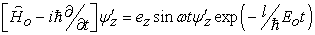(1)

Neglecting the exponent with a common factor of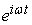and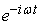which are linearly independent results from the solution of above equation to be of the form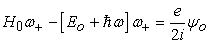(2a)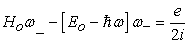(2b)

Asandare not eigenvalue ofsince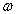is not absorption frequency, the related eigen function can be expanded to be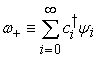(3)

This enables us to write that(4)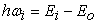(5)

Since the electric field component is said to be partially polarized in the molecules of oil, the field in operation here is from applied potential difference given as(6)

In a pair of parallel metal plate as in the case of our experiment, the stored electrostatic energy in the polarized dielectric shows that surface tension,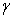of any liquid is related to electric field by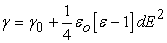(7a)

This is invariably the theoretical relation between surface tension and the applied potential difference across the plates which in terms of contact angle is given as(7b)

Where c is the capacitance, and,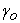is the final and initial surface tension while d is the separation between the two parallel plates which contains the dielectric material. [2, 12] Equations (1) is known as Lippmann equation while equations (5 and 7) were derived by combining the Young equation with the assumption that the change in surface tension at the sold/liquid interface is the energy stored in a capacitor across the dielectric. In terms of liquid ascent, equation (6) can be written as(8)

Since(9)(10)

Considering the fact that a pair of parallel metal plates stored electrostatic energy in polarized dielectric, the liquid ascent can be written as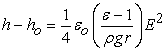(11)

From this theoretical framework deduction, non-liearity induced electric field term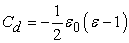is observed.

### 3. Experimental Procedure

The experiment was carried out by setting up a pair of horizontal metal plates measuring 14mm x 10mm5mm with 1mm as distant of separation between them filled with Perspex as dielectric material. Two holes are made in between the center of the metal on top for the purposes of insertion of capillary tube and the other hole serves as an avenue through which air is expelled and also for pouring of palm oil as in Figure 1 Electrical contacts provided at the appropriate terminals enable connection to a high-voltage D.C source which read using a sensitive digital avommeter while the liquid ascent is read using the a traveling microscope at an interval as DC source is adjusted. The liquid is well filtered in order to limit an abrupt change in the liquid level in the capillary tube. As the experiment went on, the applied potential was carefully checked to avoid dielectric breakdown in the set-up. The potential difference across the plates is read from the avometer while the liquid ascent is read using traveling microscope. The liquid is well filtered in order to reduce the probability of abrupt change in the liquid level in the capillary. This is because the maximum applied field in the experimental set-up is influenced by electrophoresis than dielectric breakdown

### 4. Result and Discussion

Figure one presents the graph of variation of liquid ascent in the capillary as a function of applied voltage for this experiment performed under ambient temperature from where it clearly obvious that the graph itself does not exhibit a linear as already observed by  relation accept within the threshold range which is identified between 0.00-50volt which led to maximum liquid ascent of 119x10-2m. This according to theoretical report is expected due some unobservable factor such as change in the surface tension at the solid / liquid interface that often result from the alteration of liquid –solid interfacial energy as explained by Berge. [2, 13] Invariably, it has also been observed that the applied potential tends not vary with contact angle a particular known as saturation potential. .

Figure 2 depicts the comparative analysis of the predicted and analytical results in relation to the applied potential and capillary rise using Young / Lippmann equation for surface tension while that of the logarithm of the applied field and the liquid ascent is shown in Figure 3 which did not show any significant indication of linearity of the system. However it was also observed that from this theoretical analysis there was no linear relation between the two variables as indicated in the predicted result. The analytical graph has the same values at only two points; 1.75x103V and 3.20x103V. The observation to be noted in these results was that there is a required threshold voltage in order to obtain an appreciable liquid ascent during the experiment.

Figure 1. Graph of Liquid ascent h/10-5 m as a of electric Field Ex/10Vm-1
Figure 3. the logarithm of liquid ascent as a function of Logarithm of the Electric Field

### References

  E Colgate; H.Matsumoto; J Vac Sci. Technol., (1990), An investigation of ectrowetting- based microstructure J.Voc. Sci. Technol. A8, 3625-3633In article CrossRef  Berge, B (1993) Electrocapilarity and wetting of insulator film by water, C.R Acad. Sci. Paris Ser II., (1993), 317, 157-163In article  Pollack; M.G and Fair R.B.,; A.D Shenderov, (2000), Electrowetting –based actuation of Liquid droplet for microfluidic application, Appl. Phys. let. 77, 1725-1726.In article  Prins, M.; Welters W.J and Weekamp W.J. (2001), Fluid controlling multichannel structures by electrocapillarity pressure, Science 291, 277-280.In article CrossRef  Lienemann J, Greiner A.; Korvink J.G., (2006), Modeling Simulation, and optimization of Electrowetting, IEEE, Tran. Comp-Aided Des Intgr. Cir. Syst., 25, 234-247.In article  P.Zhou; C.W.Wu, Micro and Nanosystem, (2009), 1, 57-62In article CrossRef  X.Shan; H. (1993), Lattice Boltzmann simulating flows with multiple phase and components Phys. Rev. E, 47, 1815-1819In article CrossRef  Asberg, A. J, Kponen, A, Merikoski, D and Timnen, J, (2002),lattice Boltzmann simulation of Capillary rise dynamics, J.Stat.Phys 107, 143-153In article CrossRef  H.Li; J.Li; Y. Chen; H.Fang, Comput. Phys. (2007),Lattice Boltzmann model simulation of elrctrowetting-on- dielectric in a rough wall channel. Compt. Science, http://arcxiv.org/abs/0708.4182vIn article  Haung,H, Thome D.T, Schaap, M.G and Sukop M.C, (2007), Propsed approximation for contact angles in Shan-and Shen-typemulticomponet multiphase lattice Boltzmann models, Phys. Rev E, 76, 066701In article CrossRef  Lin, P, (1978), “Some Electrocapiillary-Type Behaviour of Gold Electrode, J. Electrochem. Soc; 194, pp 1077-1098In article CrossRef  Kata, E (1991). Electrocapillarity in dielectrics, MacMillan, India Press, Madras.In article  Chen, J.H and Hsieh, W.H,. (2006), Electrowetting-induced capillary flow in a parallel-plate Channel, J. Colloid. Interface Sci., (2006), 296, 276-283.In article CrossRef  Kang K.H.; Kangn I.S and Lee,C.M, (2003), Wetting Tension Due to Coulombic Interaction in Charge- Related Wetting phenomena Langmuir Vol. 19, No. 13, 2003, 548-513.In article CrossRef